# Working with Numbers in JavaScriptNumbers and mathematics come into most scripts at some point, be it in the form of the simple arithmetic required to add up prices and work out sales tax, the process of generating and using random numbers, or the more complex mathematics involved animation.

Numbers and mathematics come into most scripts at some point, be it in the form of the simple arithmetic required to add up prices and work out sales tax, the process of generating and using random numbers, or the more complex mathematics involved animation.

In this article we’ll meet the basic constructs for number crunching in JavaScript, and look at some fairly simple, but useful snippets.

### Doing Math in JavaScript

JavaScript provides syntax for basic arithmetic, as well as a range of properties and methods for performing more complex tasks.

There are five arithmetic operators in JavaScript, each of which is represented by a special character: multiplication (*), division (/), addition (+), subtraction (-), and modulus(%).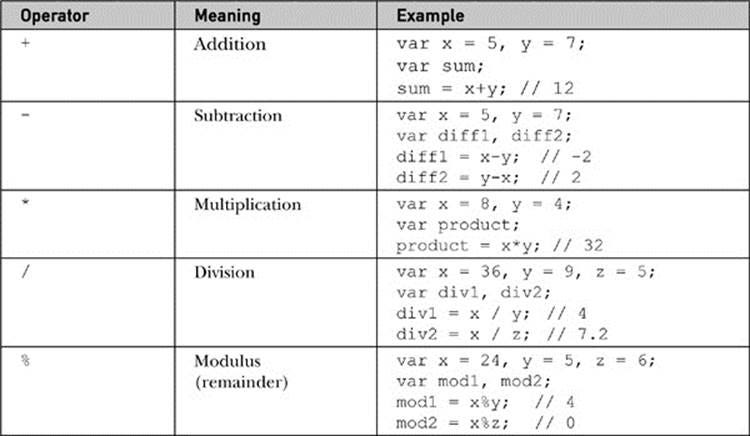Math Operators

In addition to these basic operators, JavaScript has a built-in Math object that provides a range of mathematical methods and properties. The most useful methods are:

Math.ceil : rounds a number upwards, so Math.ceil(8.23) returns 9

Math.floor : rounds a number downwards, so* Math.floor(23.8)* returns 23

Math.round : rounds the number to the nearest integer, so *Math.round(8.3) *returns 8, while Math.round(2.8) returns 3

Math.pow : raises one number to the power of another so Math.pow(2,3)returns

Math.sqrt : returns the square root of a number do *Math.sqrt(9) *returns 3

Math.random : returns a pseudorandom number between zero and one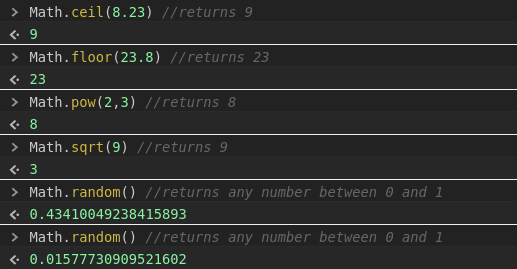### Rounding a Number to x Decimal Places

In some applications, you may need to round the result to a certain number of decimal places. For instance, you may want to display temprature values in 0.1 degree increments, even you though your script has access to more precise values.

So the question is how to do it?

We can write a function that will round to any number of places, as follows: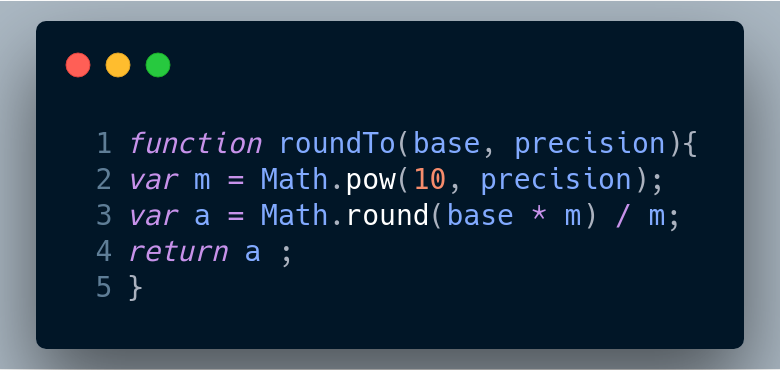JavaScript function to round any numbers

So, for example, if we started with a number like n = 3.492867, we could call roundTo(n, 0) to get 3 or roundTo(n, 2) to get 3.49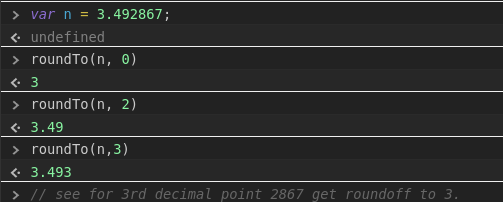### Creating and Constraining Random Numbers

Random numbers can be used as the basis for any task that needs to have a random or semi-random aspect.

We already know how to generate a random number between 0 and 1 by using Math.random() function but what if we need random numbers between 0-100 or maybe 8-23 or 23-1995 etc.

Well there is a solution

We can obtain a random integer within specified limits using a combination of the Math object’s random and round methods, which we saw in “Doing Math in JavaScript”.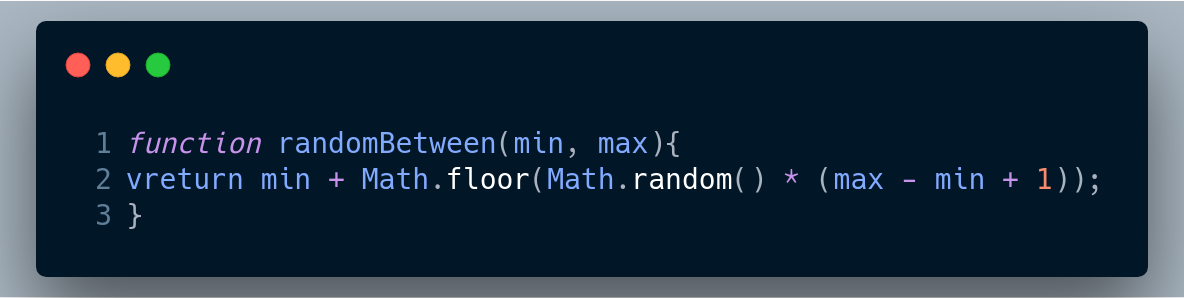function to generate random number between any two numbers.

so, for example we could generate a number between 0 and 100 by calling randomBetween(0, 100), or a number between 8 and 1995 by calling radomBetween(8, 1995).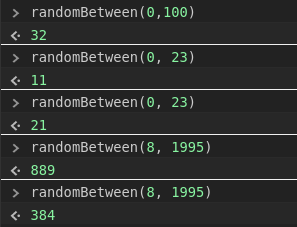using randomBetween() function to get random numbers.

### Converting a Number to a String

Once you’ve finished a calculation, you might want to turn the output into something more readable, such as formatting a value to represent currency.To do this we must convert the number to a string.

The most direct means of converting a number to a string is to built -in String constructor function: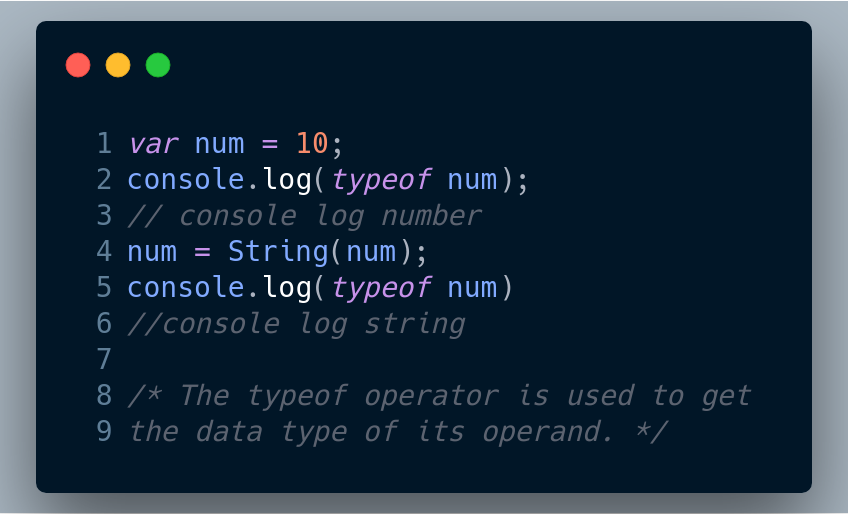converting number to string using built-in String constructor

You can also use toString method, which is provided for every number value in JavaScript: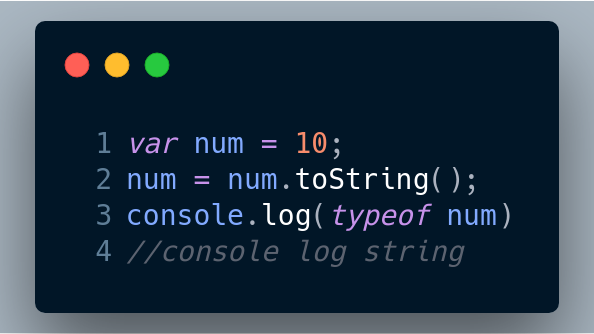converting string using toString() method

Another useful technique is string concatenation (joining strings together), which returns a string even if some of the input values are numbers: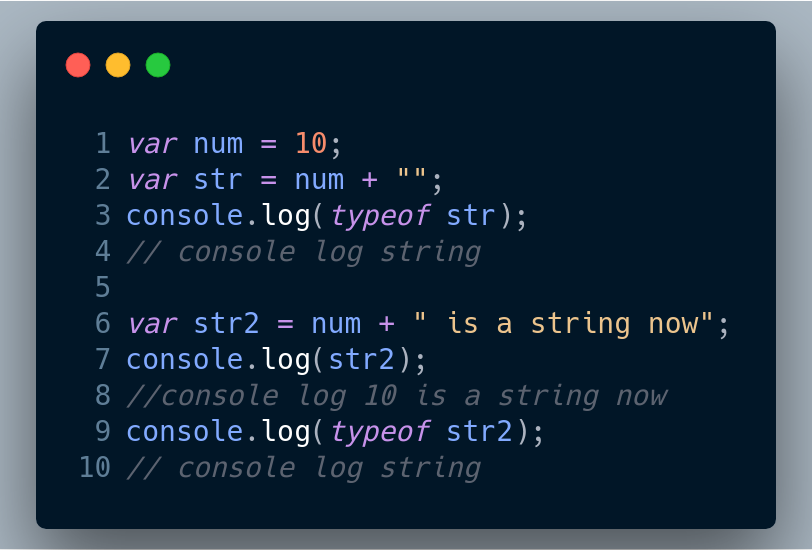string concatenation

### Converting a String to a Number

Like Number to String, conversion of String to a Number is also possible.

In general, you can treat a JavaScript string that contains a number as if it were a number, and JavaScript will Perform the string-to-number conversion for you automatically. But sometimes you need to extract a number from a string, or exercise more control over how the conversion is done.

The most direct means of converting a string to a number is to use the built-in Number constructor function: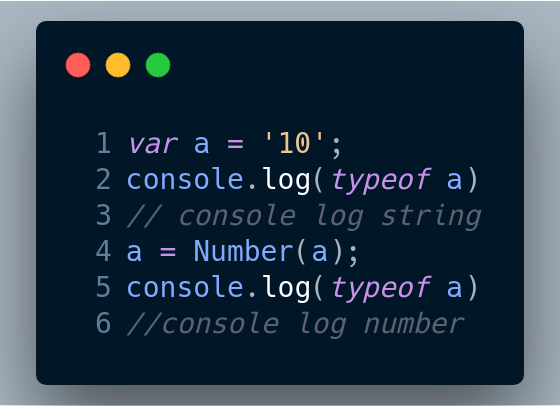conversion using built-in Number constructor function

Another handy technique is to use tha parseInt and ParseFloat functions, which will attempt to find and return an integer or decimal number at the start of a string.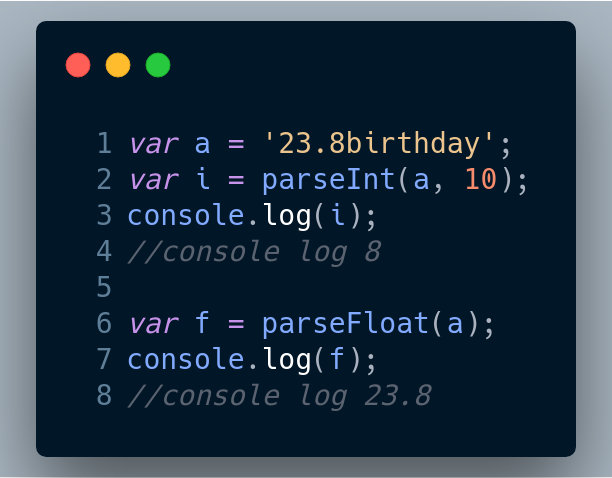conversion using parseInt and parseFloat

*note : *If the first character of the input is not a digit or some other numerical character , these functions will not be ale to return a number. In such cases. they’ll return the special value NaN (Not a Number).

## The essential JavaScript concepts that you should understand

The essential JavaScript concepts that you should understand - For successful developing and to pass a work interview

## Data Types In JavaScript

JavaScript data types are kept easy. While JavaScript data types are mostly similar to other programming languages; some of its data types can be unique. Here, we’ll outline the data types of JavaScript.

## JavaScript Memory Management System

The main goal of this article is help to readers to understand that how memory management system performs in JavaScript. I will use a shorthand such as GC which means Garbage Collection. When the browsers use Javascript, they need any memory location to store objects, functions, and all other things. Let’s deep in dive that how things going to work in GC.

## Create a Line Through Effect with JavaScript

In this post we are going to create an amazing line through effect, with help of CSS and lots of JavaScript. So, head over to your terminal and create a folder LineThroughEffect. Create three files -index.html, main.js and styles.css inside it.

## Grokking Call(), Apply() and Bind() Methods in JavaScript

In this article, we will have a look at the call(), apply() and bind() methods of JavaScript. Basically these 3 methods are used to control the invocation of the function.